# Factors Affecting the Magnitude Of Δo: - Coordination Chemistry Notes | Study Inorganic Chemistry - Chemistry

## Chemistry: Factors Affecting the Magnitude Of Δo: - Coordination Chemistry Notes | Study Inorganic Chemistry - Chemistry

The document Factors Affecting the Magnitude Of Δo: - Coordination Chemistry Notes | Study Inorganic Chemistry - Chemistry is a part of the Chemistry Course Inorganic Chemistry.
All you need of Chemistry at this link: Chemistry

Factors Affecting Magnitude of ∆o:

There are several factors which affect the magnitude of crystal field splitting (∆o) of d orbitals.

• Oxidation State of Metal Ion: The higher the oxidation state of metal ion, the greater will be the magnitude of crystal field splitting. For example, the values of crystal field splitt ing for Co2+ and Co3+ are given below.

o for [Co(H2O)6] 2+ = 9200 cm–1
And ∆o for [Co(H2O)6]3+ = 20760 cm–1

This is because; the higher the o.s. of metal causes the ligands to approach more closely to it and, therefore, the more repulsion which causes more splitting.

• Nature of Ligands: The ligands are of two types, strong field ligands and weak field ligands. Generally, carbon and nitrogen donor ligands are considered as strong field ligands whereas oxygen and halogen donor ligands are termed as weak field ligands. The weak field ligands causes the small splitting because of low attraction (and therefore less repulsion) whereas strong field ligands causes more splitting due to strong attraction (therefore strong repulsion). The common ligands have been arranged in order of their increasing crystal field splitting power and this order is known as Spectrochemical Series.

CO > CN > R3P > C6H5, CH3 > NO2 > Phen, bpy > NH2OH > SO32–, en > Py, NH3 > gly > NCS > H2O > O2– > C2O42–, > C2H5OH > OH > urea > F > N3– > Cl > SCN > S2– > Br > I

The order of field strength of the common ligand is independent of the nature of the metal cation and geometry of the complex. Usually,

Carbon donor > N-donor > O-donor > X-donor

•  No. of d-electrons: For a given series of transition elements (e.g. 3d series), in complexes having metal cation with the same o.s., the magnitude of ∆o decrease with increase in no. of d electrons. For example, the crystal field splitting values for the following metal complexes are given below:
for [Co (H2O)6]2+ = 9200 cm–1 (3d7)
o for [Ni (H2O)6]2+ = 8500 cm–1 (3d8)

This is due to the fact that the higher no. of d electrons prevents the ligands to come closer to the metal cation.

• Nature of the Series (n) of d Orbitals: In case of complexes having metal cations with same o.s. and same no. of d electrons, the magnitude of for analogous complexes within a given group increases from 3d to 4d to 5d.
For example,
For [Co (NH3)6]3+, ∆o = 23000 cm–1 (3d)
For [Rh (NH3)6]3+, ∆o = 34100 cm–1 (4d)
For [Ir (NH3)6]3+, ∆o = 41200 cm–1 (5d)

This is because, on moving to 3d to 4d to 5d, the size of d orbitals increases and electron density decreases in them. Therefore the attraction increases between the d orbital and ligands. Consequently, the ligands approach more closely to the metal cation causes increase in crystal field splitting.

•  No. of Ligands: The magnitude of crystal field splitting increases with increase of the no. of ligands. For example,

o > ∆t

High Spin and Low Spin Complexes

We saw how to calculate the CFSE of complexes having metal ion of d1, d2 and d3 configurations. Let’s discuss how to calculate CFSE of higher d electron metal complexes. For a d4 ion, two arrangements are available: the four electrons ma y occupy the t 2g set wit h the configuration t2g4eg0, or may singly occupy four d orbitals i.e. t2g3eg1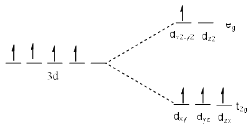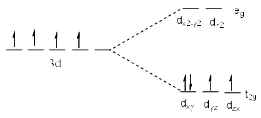High spin configuration                                     Low spin configuration

First configuration is called high spin and the second is called the low spin.
High spin complexes or spin free complexes are formed in the presence of weak field ligands (oxygen and halogen donor ligands) while low spin or spin paired complexes are formed in the presence of strong field ligands (carbon and nitrogen donor ligands). In low spin complexes we forcibly pair up electrons. Hence pairing energy is introduced while calculating CFSE of low spin complexes according to the ground state configuration.
For example, the occupation of the 3d orbitals in weak and strong field Fe3+ (d5) complexes is shown below.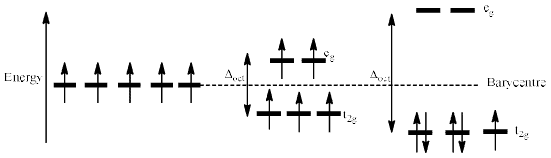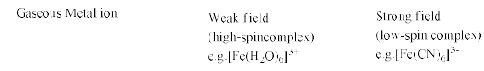Pairing Energy: The energy required to pair up two unpaired electrons in a orbital is called pairing energy. It is denoted by P.
If ∆o > P, low spin complexes are formed.
If ∆o < P, high spin complexes are formed.
If ∆o = P, high spin and low spin complexes exist in equilibrium.

CFSE in High and Low Spin Complexes:

d4 Configuration:

 High Spin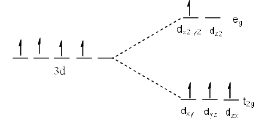CFSE = nt2g × (-0.4∆o) + neg × (0.6∆o) = 3 × (-0.4∆o) + 1 × (0.6∆o)CFSE = -0.6∆o Low Spin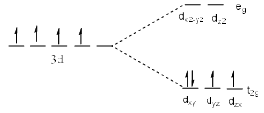CFSE = nt2g × (-0.4∆o) + neg × (0.6∆o)                                                                      = 4 × (-0.4∆o) + 0 × (0.6∆o) + P                                                                                                          CFSE = -1.6∆o + P

d5 Configuration:

 High Spin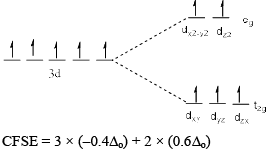CFSE = 0 Low Spin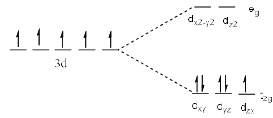CFSE = 5 × (–0.4∆o) + 0 × (0.6∆o) + 2PCFSE = –2.0 ∆o + 2P

d6 Configuration:

 High Spin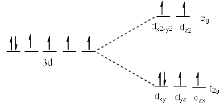CFSE = 4 × (–0.4∆o) + 2 × (0.6∆o)CFSE = –0.4∆o Low SpinCFSE = 6 × (–0.4∆o) + 0 × (0.6∆o) + 2P CFSE = –2.4∆o + 2P

Pairing energy is only taken for those electrons which are not paired in the ground state and we are forcibly pairing up them in the complex.

d7 Configuration:

 High Spin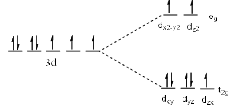CFSE = 5 × (–0.4∆o) + 2 × (0.6∆o) CFSE = –0.8∆o Low Spin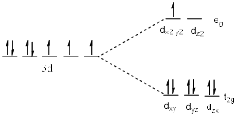CFSE = 6 × (–0.4∆o) + 1 × (0.6∆o) + P CFSE = –1.8∆o+ 2P

d8 Configuration: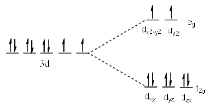CFSE = 6 × (–0.4∆o) + 2 × (0.6∆o)
CFSE = –1.2∆o

d9 Configuration: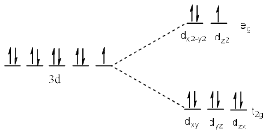CFSE = 6 × (–0.4∆o) + 3 × (0.6∆o)
CFSE = –0.6∆o

d10 Configuration: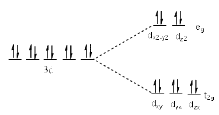CFSE = 6 × (–0.4∆o) + 4 × (0.6∆o) = 0

The following table summarises the CFSE values of all the electronic configurations in an octahedral complex.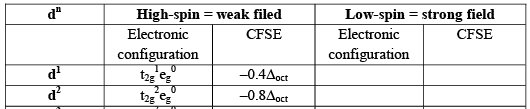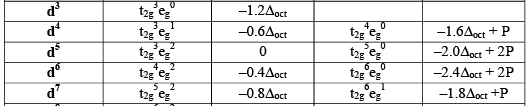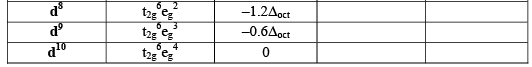The document Factors Affecting the Magnitude Of Δo: - Coordination Chemistry Notes | Study Inorganic Chemistry - Chemistry is a part of the Chemistry Course Inorganic Chemistry.
All you need of Chemistry at this link: ChemistryUse Code STAYHOME200 and get INR 200 additional OFF

## Inorganic Chemistry

49 videos|71 docs|16 tests

Track your progress, build streaks, highlight & save important lessons and more!

,

,

,

,

,

,

,

,

,

,

,

,

,

,

,

,

,

,

,

,

,

;